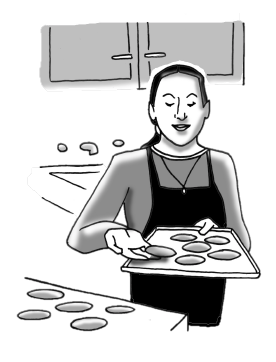### Home > CC3MN > Chapter 4 > Lesson 4.1.6 > Problem4-63

4-63.Ms. B is making snickerdoodle cookies. Her recipe uses one and a half teaspoons of cinnamon to make two dozen cookies. If she needs to make thirteen dozen cookies to give one cookie to each of her students, how much cinnamon will she need? There are three teaspoons in one tablespoon. How many tablespoons and teaspoons will Ms. B need?

Use proportions to solve this problem. Assign a variable to the unknown number of teaspoons of cinnamon.
For this case, you can use the variable $n$

$\frac{ 1 \frac{ 1 }{2 } \text{ teaspoons}}{ 2\text{ dozen cookies}}= \frac{ n\text{ teaspoons}}{ 13\text{ dozen cookies}}$

Multiply both sides by $2$.

$\frac{ 1 \frac{ 1 }{2 } }{2 }= \frac{ n }{13 }$

Multiply both sides by $13$.

$1 \frac{ 1 }{2 } = \frac{ 2n }{13 }$

Divide by $2$ on both sides.

$19.5=2n$

Remember to use the conversion factor to determine how many tablespoons and teaspoons Ms. B will need.

$n=9.75 \text{ or }9 \frac{3}{4} \text{ teaspoons}$